# You can create a simple anonymous function eigenvalues sum by the following line of code: and similarly, define eigenvalues product by Create at least five matrices and compute their eigenvalues sum,...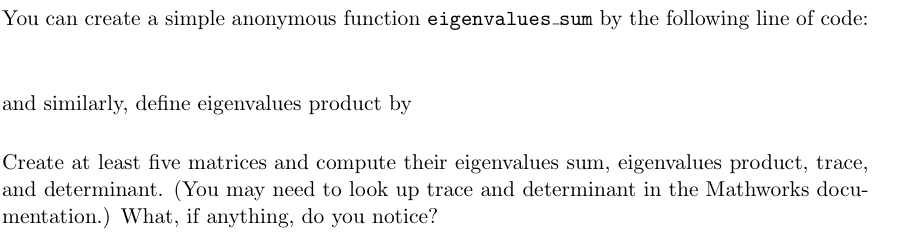You can create a simple anonymous function

eigenvalues

sum

by the following line of code:

and similarly, define eigenvalues product by

Create at least five matrices and compute their eigenvalues sum, eigenvalues product, trace,

and determinant. (You may need to look up trace and determinant in the Mathworks docu-

mentation.) What, if anything, do you notice?

MATLAB: I need the code for everything in the picture, not just the bottom paragraph. Thanks!

You can create a simple anonymous function eigenvalues sum by the following line of code and similarly, define eigenvalues product by Create at least five matrices and compute their eigenvalues sum, eigenvalues product, trace, and determinant. (You may need to look up trace and determinant in the Mathworks docu mentation.) What, if anything, do you notice?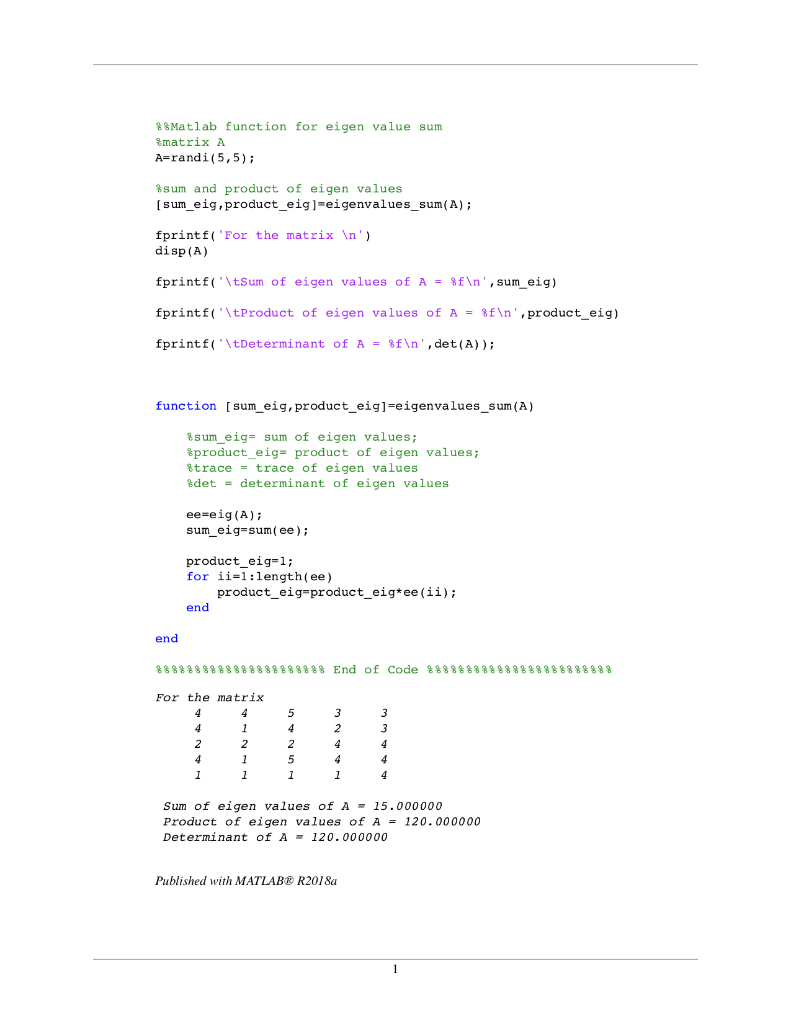%%Matlab function for eigen value sum
%matrix A
A=randi(5,5);

%sum and product of eigen values
[sum_eig,product_eig]=eigenvalues_sum(A);

fprintf('For the matrix \n')
disp(A)

fprintf('\tSum of eigen values of A = %f\n',sum_eig)

fprintf('\tProduct of eigen values of A = %f\n',product_eig)

fprintf('\tDeterminant of A = %f\n',det(A));

function [sum_eig,product_eig]=eigenvalues_sum(A)

%sum_eig= sum of eigen values;
%product_eig= product of eigen values;
%trace = trace of eigen values
%det = determinant of eigen values

ee=eig(A);
sum_eig=sum(ee);

product_eig=1;
for ii=1:length(ee)
product_eig=product_eig*ee(ii);
end

end

%%%%%%%%%%%%%%%%%%%%%% End of Code %%%%%%%%%%%%%%%%%%%%%%%%

##### Add Answer of: You can create a simple anonymous function eigenvalues sum by the following line of code: and similarly, define eigenvalues product by Create at least five matrices and compute their eigenvalues sum,...
Similar Homework Help Questions
• ### You should test out your script using the following matrices. 2 3 1 2 3 1 C D- 3 2 B- A- -3 8 4 8 2 4 4 1 You may n...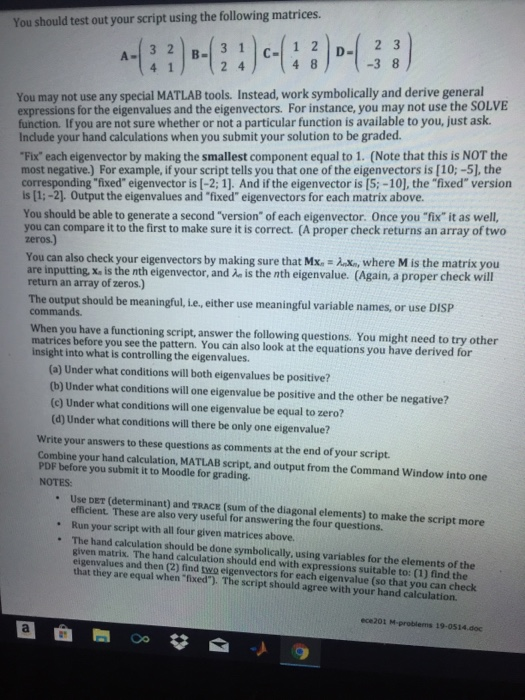You should test out your script using the following matrices. 2 3 1 2 3 1 C D- 3 2 B- A- -3 8 4 8 2 4 4 1 You may not use any special MATLAB tools. Instead, work symbolically and derive general expressions for the eigenvalues and the eigenvectors. For instance, you may not use the SOLVE function. If you are not sure whether or not a particular function is available to you, just ask Include your hand...

• ### Can I please get some help with this?? It's matlab. Code 1 1) Define a function as [NE]- nb_elemt24( n1,n2). 2) Define two vectors A and B by randi(10, [1,n1]) and randi(10, [1,n2]), respectively...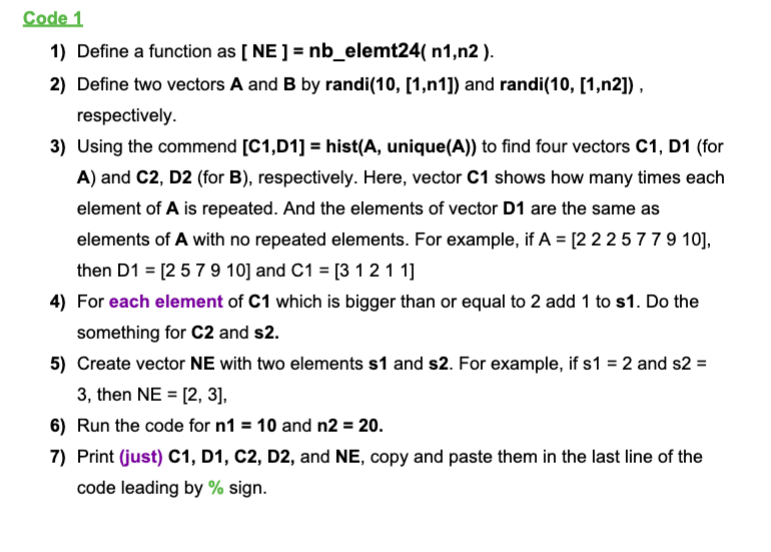Can I please get some help with this?? It's matlab. Code 1 1) Define a function as [NE]- nb_elemt24( n1,n2). 2) Define two vectors A and B by randi(10, [1,n1]) and randi(10, [1,n2]), respectively. 3) Using the commend [C1,D1] hist(A, unique(A)) to find four vectors C1, D1 (for A) and C2, D2 (for B), respectively. Here, vector C1 shows how many times each element of A is repeated. And the elements of vector D1 are the same as elements of...

• ### In Python and in one file please. (Simple functions with an expressions) Create a function called load_inventory(filenam...

In Python and in one file please. (Simple functions with an expressions) Create a function called load_inventory(filename). The filename argument in this case specifies the name of a file that contains all the inventory/product information for the store, including product names, descriptions, prices, and stock levels. This function should clear any information already in the product list (i.e., a fresh start) and then re-initialize the product list using the file specified by the filename argument. You can structure your file...

• ### How do i write the pseudocode for this java code? First, write out pseudocode, and then create a program to help you by accomplishing the following tasks: :  Use command line interface to ask the use...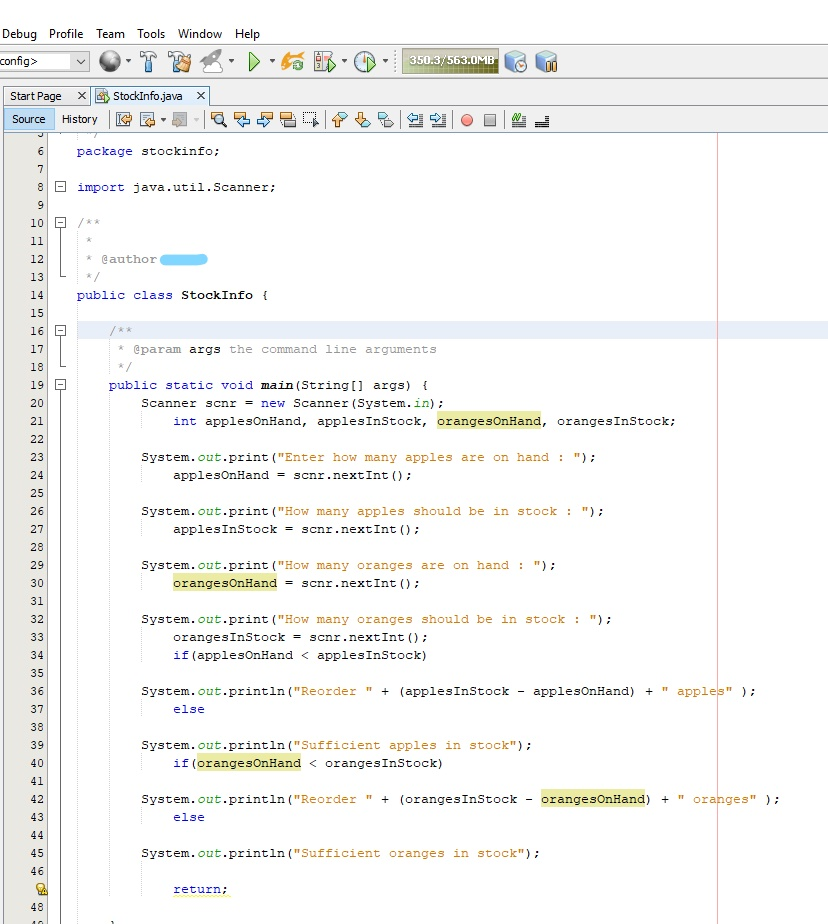How do i write the pseudocode for this java code? First, write out pseudocode, and then create a program to help you by accomplishing the following tasks: :  Use command line interface to ask the user to input the following. ○ How many apples are on hand ○ How many apples should be in stock ○ How many oranges are on hand ○ How many oranges should be in stock  Perform an operation to determine how many of...

• ### Create a program that performs the following operations: Prompt for and accept a string of up...

Create a program that performs the following operations: Prompt for and accept a string of up to 80 characters from the user. • The memory buffer for this string is created by: buffer: .space 80 # create space for string input • The syscall to place input into the buffer looks like: li \$v0, 8 # code for syscall read_string la \$a0, buffer # tell syscall where the buffer is li \$a1, 80 # tell syscall how big the buffer...

• ### Write a C++ program for the instructions below. Please read the instructions carefully and make sure they are followed correctly.   and please put comment with code! Problem:2 1. Class Student Create...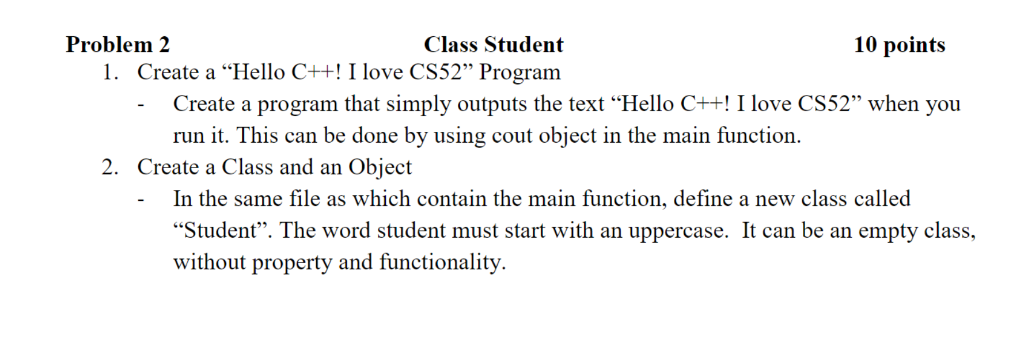Write a C++ program for the instructions below. Please read the instructions carefully and make sure they are followed correctly.   and please put comment with code! Problem:2 1. Class Student Create a "Hello C++! I love CS52" Program 10 points Create a program that simply outputs the text Hello C++!I love CS52" when you run it. This can be done by using cout object in the main function. 2. Create a Class and an Object In the same file as...

• ### Hi, could you post solutions to the following questions. Thanks. 2. (a) Let V be a vector space on R. Give the definition of a subspace W of V 2% (b) For each of the following subsets of IR3 sta...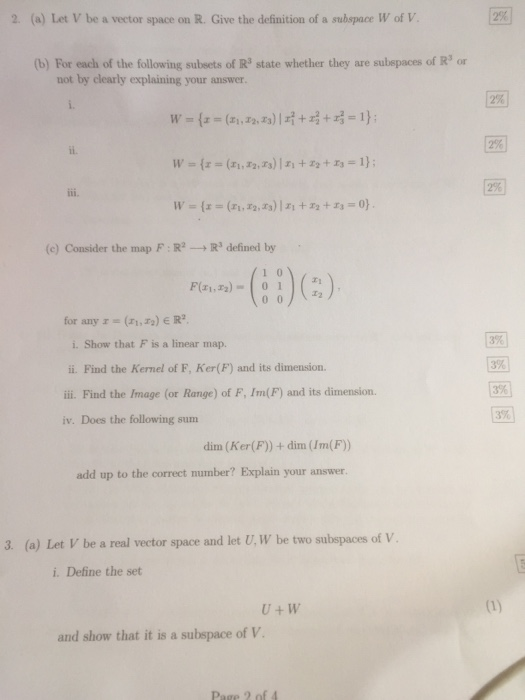Hi, could you post solutions to the following questions. Thanks. 2. (a) Let V be a vector space on R. Give the definition of a subspace W of V 2% (b) For each of the following subsets of IR3 state whether they are subepaces of R3 or not by clearly explaining your answer. 2% 2% (c) Consider the map F : R2 → R3 defined by for any z = (zi,Z2) E R2. 3% 3% 3% 3% i. Show that...

• ### SQL CODE – System Variables System variables can be set via the command line. Please write a sequence of statements to: Set safe updates to false for the session. Do the following at the global level...

SQL CODE – System Variables System variables can be set via the command line. Please write a sequence of statements to: Set safe updates to false for the session. Do the following at the global level: Set the maximum number of connections to 45. Set the threshold for long running queries to 7 seconds – do this at the global level. Set logs to expire when they are more than 3 days old Write the commands to display the value...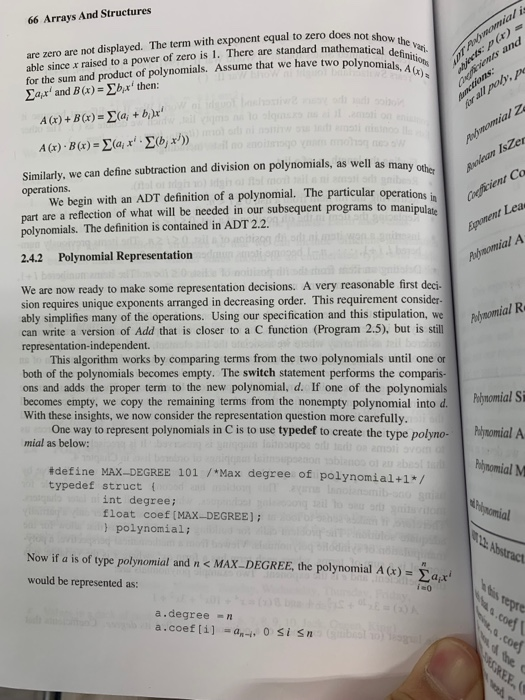The polynomial addition C function of Program 2.6 padd is the code when the polynomial is used to arrange the polynomial in the two arrangement methods of the polynomial described in the text 2.4.2. For the remaining method, when the expression polynomial is arranged by a coefficient, create a polynomial addition C function padd() corresponding to Program 2.6. 66 Arrays And Structures are zero are not displayed. The term with exponent equal to zero does not shouw able since x...